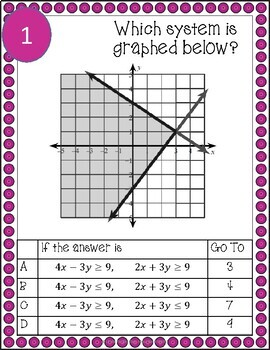# Systems of Linear Inequalities Treasure HuntSubject
Resource Type
File Type

PDF

(2 MB|30 pages)
Product Rating
Standards
• Product Description
• StandardsNEW

Need to get students out of their seats talking and working with others? A Treasure Hunt is the answer. In this fun activity, students will review systems of linear inequalities by graphing, identifying solutions and identifying shading.

The 12 problem pages are posted either around the room or in stations. Students work in groups and can start at any place, once they solve the problem, they are directed to the next page to go. Students with an error find they they have been misdirected to the wrong place and work together to find their mistake and get back on track.

Teacher can check individual answer sheets or play a stand up/sit down game once students are finished. Teacher calls out one of the answers and students find it on their answer sheet and then teacher calls out the next answer. Students who have this answer remain standing, if not they sit down. This gives teacher feedback on which problems gave the most difficulty. Answer key is included as well as student worksheet to show their work.

This is perfect for your Algebra class and a great review before a quiz or test.

Explain why the 𝘹-coordinates of the points where the graphs of the equations 𝘺 = 𝘧(𝘹) and 𝘺 = 𝑔(𝘹) intersect are the solutions of the equation 𝘧(𝘹) = 𝑔(𝘹); find the solutions approximately, e.g., using technology to graph the functions, make tables of values, or find successive approximations. Include cases where 𝘧(𝘹) and/or 𝑔(𝘹) are linear, polynomial, rational, absolute value, exponential, and logarithmic functions.
Understand that the graph of an equation in two variables is the set of all its solutions plotted in the coordinate plane, often forming a curve (which could be a line).
Solve a simple system consisting of a linear equation and a quadratic equation in two variables algebraically and graphically. For example, find the points of intersection between the line 𝘺 = –3𝘹 and the circle 𝘹² + 𝘺² = 3.
Solve linear equations and inequalities in one variable, including equations with coefficients represented by letters.
Explain each step in solving a simple equation as following from the equality of numbers asserted at the previous step, starting from the assumption that the original equation has a solution. Construct a viable argument to justify a solution method.
Total Pages
30 pages
Included
Teaching Duration
50 minutes
Report this Resource to TpT
Reported resources will be reviewed by our team. Report this resource to let us know if this resource violates TpT’s content guidelines.